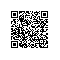# 《JavaScript应用程序设计》一一2.7 变量提升

### 2.7 变量提升

JavaScript的执行环境构建分为声明阶段和执行阶段。在声明阶段JavaScript引擎为所有变量与函数声明创建标识符，可以将此阶段看作是对运行环境的前期配置。到了执行阶段，函数均已被定义，但所有变量的值均未定义，例如:

var x = 1;

(function () {
console.log(x);
var x = 2;
}());


var x = 1;

(function () {
var x; // Declaration is hoisted and x is undefined.
console.log(x);
x = 2; // Initialization is still down here.
}());


test('Function declaration hoisting', function () {
function number() {
return 1;
}

(function () {
equal(number(), 2, 'Inner scope wins.');

function number() {
return 2;
}
}());

equal(number(), 1, 'Outer scope still works.');
});


test('Function declaration hoisted.', function () {
function number() {
return 1;
}

(function () {
function number() {
return 2;
}

equal(number(), 2, 'Inner scope wins.');
}());

equal(number(), 1, 'Outer scope still works.');
});


test('Function expression hoisting', function () {
function number() {
return 1;
}

(function () {
try {
number();
} catch (e) {
ok(true, 'number() is undefined.');
}

var number = function number() {
return 2;
}

equal(number(), 2, 'number() is defined now.');
}());

equal(number(), 1, 'Outer scope still works.');
});


test('Function Expression Hoisted', function () {
function number() {
return 1;
}

(function () {
var number; // Declaration initialized to undefined.

try {
number();
} catch (e) {
ok(true, 'number() is undefined.');
}

number = function number() {
return 2;
}

equal(number(), 2, 'number() is defined now.');
}());

equal(number(), 1, 'Outer scope still works.');
});